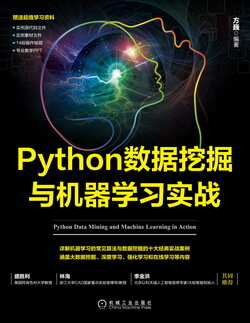# Python 数据挖掘与机器学习实战 (56)：回归分析介 3.2.1(简单线性回归分析)

$Y=a+bx\phantom{\rule{1em}{0ex}}\phantom{\rule{1em}{0ex}}\phantom{\rule{1em}{0ex}}\phantom{\rule{1em}{0ex}}\phantom{\rule{1em}{0ex}}\phantom{\rule{1em}{0ex}}\phantom{\rule{1em}{0ex}}\left(3-1\right)$$Y=a+bx\quad\quad\quad\quad\quad\quad\quad(3-1)$